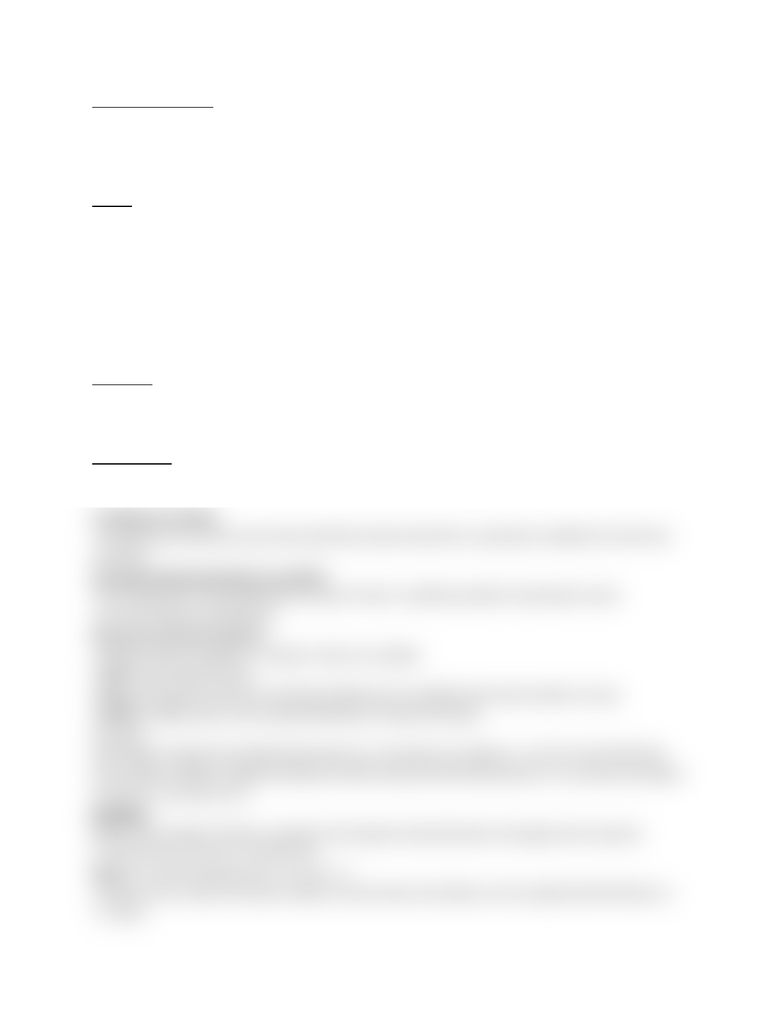Class Notes (1,100,000)
CA (630,000)
U of G (30,000)
SOAN (900)
SOAN 2120 (400)
Lecture

# SOAN 2120 Mar.21.docx

Department
Sociology and Anthropology
Course Code
SOAN 2120
Professor
Scott Schau

This preview shows half of the first page. to view the full 2 pages of the document.Quantitative Analysis
-numerical representation and manipulation of observations for the purpose of describing and
explaining the phenomena that those observations reflect
Coding in Quantitative Data Analysis-getting the data from the subjects into a format that we can
manipulate and understand
Coding
-for computers to work, you must translate your data into something that they can read
-coding schemes are guided by theory
-codebook-document that describes the location of variables w/in a data set and lists the codes
assigned to the attributes composing those variables
[i.e. a computer doesn’t understand M & F so we say that males could be 0 and females are 1]
Purposes:
-primary guide used in the coding process
-guide for locating variables and interpreting codes in the data fine during analysis
Data Entry
-data entry specialists enter the data into statistical software of Excel spreadsheet
-optical scan sheets
-sometimes it’s part of the process of data collection
Data Cleaning
Possible-code cleaning
-codes which have not been assigned to the attributes of a variable are removed
Contingency cleaning
-checking that only those cases that should have data entered for a particular variable do in fact have
such data
Univariate Statistical Analysis [1 variable]
-the examination of the distribution of cases of only 1 variable [usually for descriptive cases]
-one-way frequency distribution
Measures of Central Tendency
-measure of the “average” or “typical” value of a variable
-mode: most frequent value
-mean: the division of the sum of all the attributes of a variable by the total number of cases
-median: middle value in the ranked distribution of observed values
Formulas
odd sample: median=[(n+1)/2]th observation [i.e. if we have 11 numbers-> n=11 (n+1=12) (12/2=6)]
even sample: median= midpoint between (n/2)th and [(n/2)+1]th observation [i.e. we have 10 numbers
n=10 (n+1= 11) (11/2=5.5) ]
Examples:
below is the number of times a sample of 20 students had Kraft dinner throughout the semester
7 2 16 4 0 6 13 4 5 12 0 1 3 9 8 45 3 9 0
Mean= 7+2+16+7+4+0+6+13+4…+9 / 20 = 7.7
-interpret your results: the mean number of times these 20 students in the sample had Kraft dinner is
7.7 times